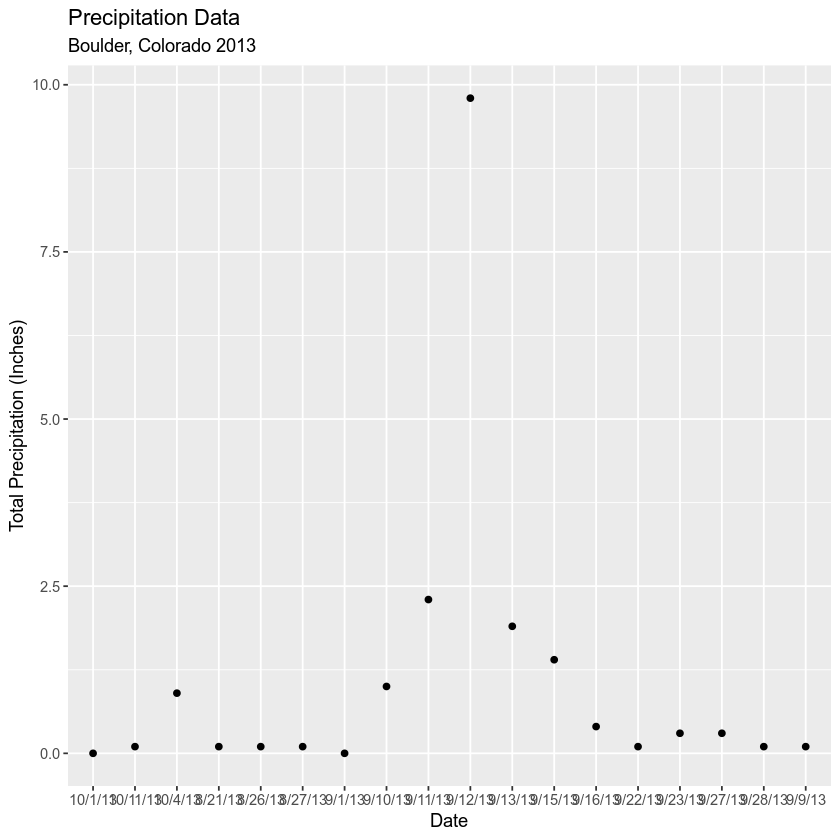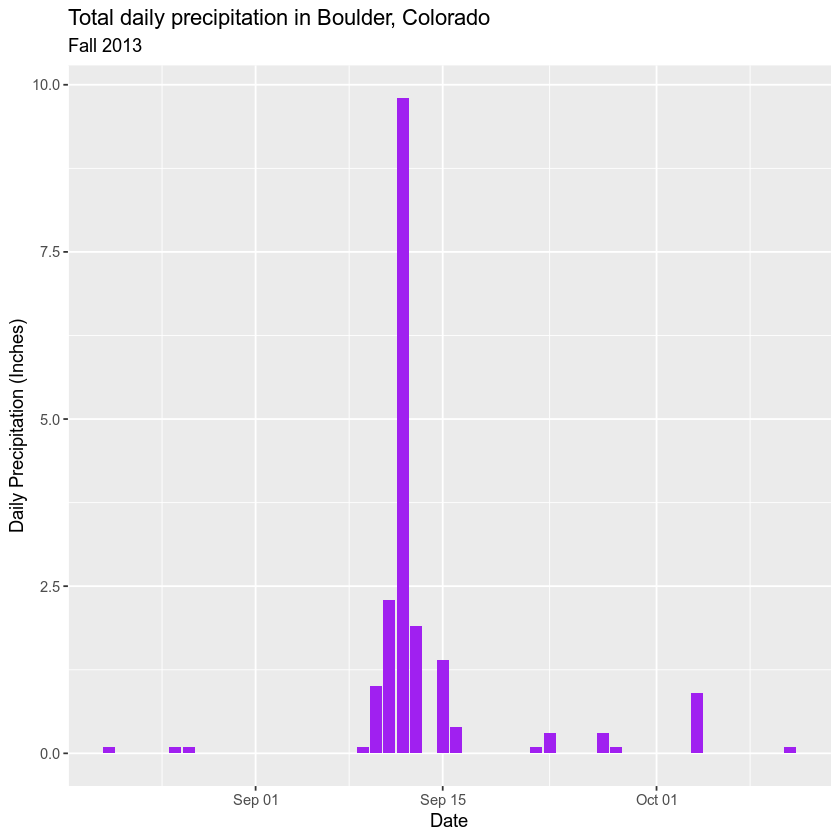# Work with Sensor Network Derived Time Series Data in R - Earth analytics course module

Welcome to the first lesson in the Work with Sensor Network Derived Time Series Data in R module. This module covers how to work with, plot and subset data with date fields in R. It also covers how to plot data using ggplot.

## Get Started with Date Formats in R

In this tutorial, you will look at the date time format - which is important for plotting and working with time series data in R.

## Learning Objectives

At the end of this activity, you will be able to:

• Convert a column in a data.frame containing dates and times to a date/time object that can be used in R.
• Be able to describe how you can use the data class ‘date’ to create easier to read time series plots in R.

## What You Need

You need R and RStudio to complete this tutorial. Also we recommend that you have an earth-analytics directory set up on your computer with a /data directory within it.

In this tutorial, you will learn how to convert data that contain dates and times into a date / time format in R.

First let’s revisit the boulder_precip data variable that you’ve been working with in this module.

# load the ggplot2 library for plotting
library(ggplot2)
options(stringsAsFactors = FALSE)
# is commented out. If you want to redownload the data, umcomment the line below.
"data/boulder-precip.csv",
method = "libcurl")

# import data

# view first few rows of the data
##    ID    DATE PRECIP TEMP
## 1 756 8/21/13    0.1   55
## 2 757 8/26/13    0.1   25
## 3 758 8/27/13    0.1   NA
## 4 759  9/1/13    0.0 -999
## 5 760  9/9/13    0.1   15
## 6 761 9/10/13    1.0   25


Next, plot the data using ggplot().

# plot the data using ggplot
ggplot(data = boulder_precip, aes(x = DATE, y = PRECIP)) +
geom_point() +
labs(x = "Date",
y = "Total Precipitation (Inches)",
title = "Precipitation Data",Notice when you plot the data, the x axis is “messy”. It would be easier to read if you only had ticks on the x axis for dates incrementally - every few weeks. Or once a month even.

Let’s look closely at the structure of the data to understand why R is placing so many labels on the x axis.

str(boulder_precip)
## 'data.frame':	18 obs. of  4 variables:
##  $ID : int 756 757 758 759 760 761 762 763 764 765 ... ##$ DATE  : chr  "8/21/13" "8/26/13" "8/27/13" "9/1/13" ...
##  $PRECIP: num 0.1 0.1 0.1 0 0.1 1 2.3 9.8 1.9 1.4 ... ##$ TEMP  : int  55 25 NA -999 15 25 65 NA 95 -999 ...


## Data Types (Classes) in R

The structure results above tell us that the data columns in your data.frame are stored as several different data types or classes as follows:

• chr - Character: It holds strings that are composed of letters and words. Character class data cannot be interpreted numerically - that is to say you can not perform math on these values even if they contain only numbers.
• int - Integer: It holds numbers that are whole integers without decimals. Mathematical operations can be performed on integers.
• num - Numeric: It accepts data that are a wide variety of numeric formats including decimals (floating point values) and integers. Numeric also accept larger numbers than int will.

### Data Frame Columns Can Only Contain One Data Class

A data.frame column can only store one type. This means that a column cannot store both numbers and strings. If a column contains a list of numbers and one letter, then the entire column will be stored as a chr (character).

Storing variables using different classes is a strategic decision by R (and other programming languages) that optimizes processing and storage. It allows:

• data to be processed more quickly & efficiently.
• the program (R) to minimize the storage size.

Remember, that you also learned about classes during class in these lessons: vectors in R - data classes

## Dates Stored as Characters

Note that the Date column in your data.frame is of class character (chr). This means that R is reading it as letters and numbers rather than dates that contain a value that is sequential.

# View data class for each column that you wish to plot
class(boulder_precip$DATE) ##  "character" class(boulder_precip$PRECIP)
##  "numeric"


Thus, when you plot, R tries to plot EVERY date value in your data, on the x-axis. This makes it hard to read. But also it makes it hard to work with the data. For instance - what if you wanted to subset out a particular time period from your data? You can’t do that if the data are stored as characters.

The PRECIP data is numeric so that variable plots just fine.

## Convert Date to an R Date Class

You need to convert your date column, which is currently stored as a character to a date class that can be displayed as a continuous variable. Lucky for us, R has a date class. You can convert the date field to a date class using the function as.Date().

When you convert, you need to tell R how the date is formatted - where it can find the month, day and year and what format each element is in.

For example: 1/1/10 vs 1-1-2010

Looking at the results above, you see that your data are stored in the format: Year-Month-Day (2003-08-21). Each part of the date is separated in this case with a -. You can use this information to populate your format string using the following designations for the components of the date-time data:

• %Y - 4 digit year
• %y - 2 digit year
• %m - month
• %d - day

Your format string will look like this: %m/%d/%y. Notice that you are telling R where to find the year (%y), month (%m) and day (%d). Also notice that you include the dashes that separate each component in each date cell of your data.

NOTE: look up ?strptime to see all of the date “elements” that you can use to describe the format of a date string in R.

# convert date column to date class
boulder_precip$DATE <- as.Date(boulder_precip$DATE,
format = "%m/%d/%y")

# view R class of data
class(boulder_precip$DATE) ##  "Date" # view results head(boulder_precip$DATE)
##  "2013-08-21" "2013-08-26" "2013-08-27" "2013-09-01" "2013-09-09"
##  "2013-09-10"


Now that you have adjusted the date, let’s plot again. Notice that it plots much quicker now that R recognizes date as a date class. R can aggregate ticks on the x-axis by year instead of trying to plot every day!

# quickly plot the data and include a title using main = ""
# use '\n' to force the string to wrap onto a new line

ggplot(data = boulder_precip, aes(x = DATE, y = PRECIP)) +
geom_bar(stat = "identity", fill = "purple") +
labs(title = "Total daily precipitation in Boulder, Colorado",
subtitle = "Fall 2013",
x = "Date", y = "Daily Precipitation (Inches)")Now, your plot looks a lot nicer!